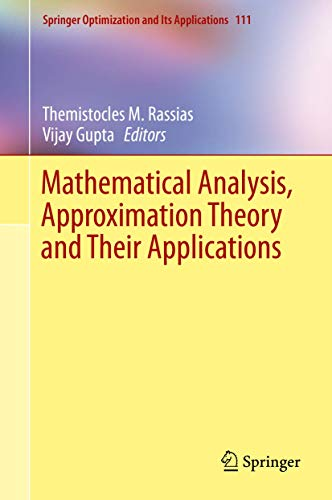# Mathematical Analysis, Approximation Theory and Their Applications: 111 (Springer Optimization and Its Applications)

0 durchschnittliche Bewertung
( 0 Bewertungen bei Goodreads )Alle Exemplare der Ausgabe mit dieser ISBN anzeigen:

DESIGNED FOR GRADUATE STUDENTS, RESEARCHERS, AND ENGINEERS IN MATHEMATICS, OPTIMIZATION, AND ECONOMICS, THIS SELF-CONTAINED VOLUME PRESENTS THEORY, METHODS, AND APPLICATIONS IN MATHEMATICAL ANALYSIS AND APPROXIMATION THEORY. SPECIFIC TOPICS INCLUDE: APPROXIMATION OF FUNCTIONS BY LINEAR POSITIVE OPERATORS WITH APPLICATIONS TO COMPUTER AIDED GEOMETRIC DESIGN, NUMERICAL ANALYSIS, OPTIMIZATION THEORY, AND SOLUTIONS OF DIFFERENTIAL EQUATIONS. RECENT AND SIGNIFICANT DEVELOPMENTS IN APPROXIMATION THEORY, SPECIAL FUNCTIONS AND Q-CALCULUS ALONG WITH THEIR APPLICATIONS TO MATHEMATICS, ENGINEERING, AND SOCIAL SCIENCES ARE DISCUSSED AND ANALYZED. EACH CHAPTER ENRICHES THE UNDERSTANDING OF CURRENT RESEARCH PROBLEMS AND THEORIES IN PURE AND APPLIED RESEARCH.

Die Inhaltsangabe kann sich auf eine andere Ausgabe dieses Titels beziehen.

Rese�a del editor:

Designed for graduate students, researchers, and engineers in mathematics, optimization, and economics, this self-contained volume presents theory, methods, and applications in mathematical analysis and approximation theory. Specific topics include: approximation of functions by linear positive operators with applications to computer aided geometric design, numerical analysis, optimization theory, and solutions of differential equations. Recent and significant developments in approximation theory, special functions and q-calculus along with their applications to mathematics, engineering, and social sciences are discussed and analyzed. Each chapter enriches the understanding of current research problems and theories in pure and applied research.

��ber diesen Titel� kann sich auf eine andere Ausgabe dieses Titels beziehen.

EUR 162,63

Versand: EUR 28,99
Von Deutschland nach USA

Versandziele, Kosten & Dauer

In den Warenkorb

### Weitere beliebte Ausgaben desselben Titels

#### Vorgestellte Ausgabe

ISBN 10: 3319810057 ISBN 13: 9783319810058
Verlag: Springer, 2018
Softcover## Mathematical Analysis, Approximation Theory and Their Applications

Verlag: Springer International Publishing (2016)
ISBN 10: 3319312790 ISBN 13: 9783319312798
Neu Hardcover Anzahl: 1
Anbieter:
AHA-BUCH GmbH
(Einbeck, Deutschland)

Buchbeschreibung Buch. Zustand: Neu. Druck auf Anfrage Neuware -Designed for graduate students, researchers, and engineers in mathematics, optimization, and economics, this self-contained volume presents theory, methods, and applications in mathematical analysis and approximation theory. Specific topics include: approximation of functions by linear positive operators with applications to computer aided geometric design, numerical analysis, optimization theory, and solutions of differential equations. Recent and significant developments in approximation theory, special functions and q-calculus along with their applications to mathematics, engineering, and social sciences are discussed and analyzed. Each chapter enriches the understanding of current research problems and theories in pure and applied research. 756 pp. Englisch. Artikel-Nr. 9783319312798

Neu kaufen
EUR 162,63
W�hrung umrechnen
Versand: EUR 28,99
Von Deutschland nach USA
Versandziele, Kosten & Dauer